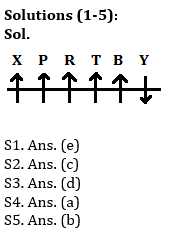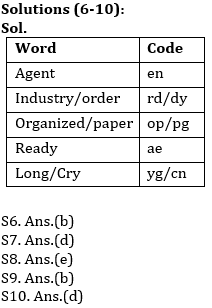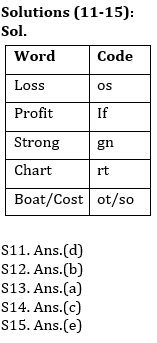Latest Banking jobs   »   Reasoning Ability Quiz For IBPS Clerk...

# Reasoning Ability Quiz For IBPS Clerk Prelims 2021- 25th August

Directions (1-5): Study the following information carefully and answer the questions given below:
Six persons are sitting in a row Some of them are facing to the north while rest are facing to South. P sits 3rd to the left of B and both does not sit at the extreme ends. The persons who sit at the extreme ends are facing opposite direction. There are two persons sit between X and T. X does not sit near to B. R sits 3rd to the right of Y, who is an immediate neighbor of B. R faces to the north. Y faces opposite direction with respect to R. Both P and T are facing in the same direction as R.

Q1. How many persons are facing to the north?
(a) Two
(b) Three
(c) One
(d) Four
(e) Five

Q2. Four of the following five are alike in a certain way and hence they form a group. Which one of the following does not belong to that group?
(a) P
(b) T
(c) Y
(d) B
(e) R

Q3. What is the position of X with respect to R?
(a) 3rd to the left
(b) 2nd to the right
(c) Immediate to the left
(d) 2nd to the left
(e) None of these

Q4. How many persons are sit right side from T?
(a) Two
(b) One
(c) Three
(d) Four
(e) None of these

Q5. Who among the following persons are sitting at extreme end?
(a) P-X
(b) X-Y
(c) P-T
(d) B-X
(e) R-Y

Directions (6-10): Study the following information carefully and answer the questions given below:
In a certain code language:
‘Agent Industry Order’ is written as ‘rd en dy’,
‘Organized Agent Paper’ is written as ‘op pg en’,
‘Industry Ready Order’ is written as ‘dy rd ae’
‘Agent Long Cry’ is written as ‘en yg cn’.

Q6. What is the code for ‘Ready’ in the given code language?
(a) rd
(b) ae
(c) dy
(d) cn
(e) None of these

Q7. If ‘Organized’ is coded as ‘pg’. What is the code for ‘Bank Paper’ in the given code language?
(a) op ae
(b) yg pg
(c) yg op
(d) op kb
(e) None of these

Q8. What is the code for ‘Industry’ in the given code language?
(a) en
(b) pg
(c) rd
(d) dy
(e) Can’t be determined

Q9. What may be the code for ‘order Smart Cry’ in the given code language?
(a) rd mr ew
(b) dy yg mr
(c) yg cn mr
(d) cn yg rd
(e) None of these

Q10. What may be the code for ‘long’?
(a) op
(b) cn
(c) yg
(d) Either (b) or (c)
(e) None of these

Directions (11-15): Study the following information carefully and answer the questions given below:
In a certain code language:
‘Loss Profit Strong’ is written as ‘os if gn’,
‘Profit Chart Strong’ is written as ‘rt gn if’,
‘Boat Profit Cost’ is written as ‘if ot so’
‘Strong Chart Profit’ is written as ‘gn rt if’.

Q11. What is the code for ‘Chart’ in the given code language?
(a) gn
(b) if
(c) ot
(d) rt
(e) None of these

Q12. If ‘Boat’ is coded as ‘so’. What is the code for ‘article cost’ in the given code language?
(a) le if
(b) ot le
(c) so gn
(d) if rt
(e) None of these

Q13. What is the code for ‘Profit’ in the given code language?
(a) if
(b) gn
(c) ot
(d) rt
(e) Can’t be determined

Q14. What may be the code for ‘Strong’ in the given code language?
(a) if
(b) rt
(c) gn
(d) so
(e) None of these

Q15. What may be the code for ‘Loss Chart cost’?
(a) so gn ot
(b) if gn ot
(c) os gn ot
(d) so gn if
(e) None of these

Solutions#### Congratulations!Union Budget 2023-24: Free PDF